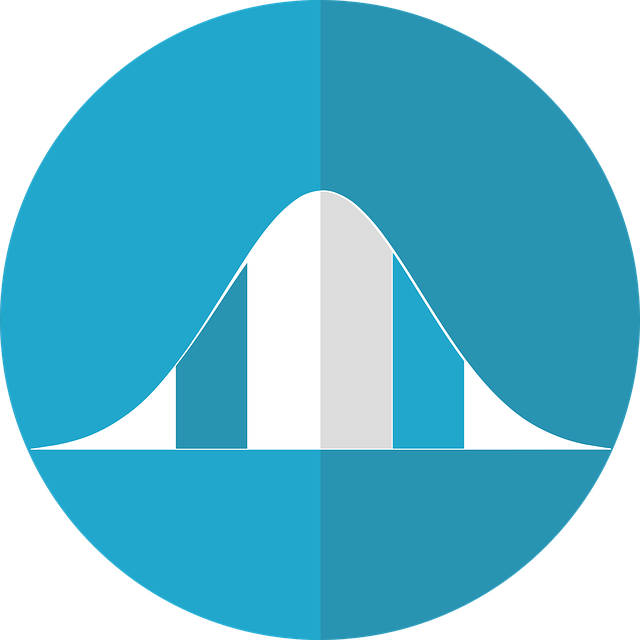# Use of Ti 84 for normal distributionLet’s talk about the use of the Ti 84 for normal distribution in this post. The graphic calculator that we refer to is the Ti 84 which most junior colleges in Singapore use for the H2 A Level Math exam.

## Two common functions of Ti 84 for normal distribution

In the normal distribution topic in H2 A Level Math Statistics, the two most important functions that we will use from the Ti 84 graphic calculator are:

• normcdf
• invnorm

## How to find normcdf in Ti 84

To find normcdf do these:

Step 1: Press [2nd]

Step 2: Press [Vars]. This will bring you to Distr.

Step 3: To go to normcdf, it is  for most Ti 84.

## When do you use normcdf from Ti 84 for normal distribution questions?

Use normcdf when you are looking for probability of a normal distribution. For example, P(X<3).

## Inputs for normcdf

There are 4 inputs required to use normcdf:

• lower: lower bound
• upper: upper bound
• μ: mean
• σ: standard deviation [note that σ = √Var(X)]

## Sample question on use of normcdf in Ti 84

### Sample question 1 on use of normcdf in Ti 84

Given X ~ N(6, 52), find P(X<4.7).

We are interested in the probability of X from negative infinity (which can be approximated to – 1099 or -E99 in the graphic calculator Ti-84) to 4.7. Hence, the lower bound is -E99 and the upper bound is 4.7.

We will input these into normcdf:

lower: -E99

upper: 4.7

μ: 6

σ: 5

P(X<4.7) = 0.397 (3 s.f.)

### Sample question 2 on use of normcdf in Ti 84

Given X ~ N(6, 52), find P(X>6.7).

We are interested in the probability of X from 6.7 (lower bound) to infinity (which can be approximated to 1099 or E99 in the graphic calculator Ti 84). Hence, the lower bound is 6.7 and the upper bound is E99.

We will input these into normcdf:

lower: 6.7

upper: E99

μ: 6

σ: 5

P(X>6.7) = 0.444 (3 s.f.)

### Sample question 3 on use of normcdf in Ti 84

Given X ~ N(6, 52), find P(3<X<5).

We are interested in the probability of X from 3 (lower bound) to 5. Hence, the lower bound is 3 and the upper bound is 5.

We will input these into normcdf:

lower: 3

upper: 5

μ: 6

σ: 5

P(3<X<5) = 0.146 (3 s.f.)

## When do you use invnorm from Ti 84 for normal distribution questions?

Use invnorm when you are looking for a value of X such that it gives a certain probability.

For example if you have P(X<x) = 0.3, what is known now is the probability, and what’s unknown now is the value of x, hence we’ll use invnorm to find this unknown value of x.

## How to find invnorm in Ti 84

To find normcdf do these:

Step 1: Press [2nd]

Step 2: Press [Vars]. This will bring you to Distr.

Step 3: To go to invnorm, it is  for most Ti 84.

## Inputs for invnorm

There are 4 inputs required for invnorm.

• area: which refers to probability (p)
• μ: mean
• σ: standard deviation
• left, center or right: tail

left tail is used when you are given P(X<a) = p

center is used when you are given P(-a<X<a) = p

right tail is used when you are given P(X>a) = p

## Sample question on use of invnorm in Ti 84 for normal distribution questions

### Sample question 1

Given X ~ N(6, 52) and P(X<a)=0.3. Find a.

To find the unknown value of x (i.e. a), for a given probability, we’ll use invnorm.

Inputs we’ll use are

area: 0.3
μ: 6
σ: 5
left tail

From Ti 84, a = 3.38 (3 s.f.)

### Sample question 2

Given X ~ N(6, 52) and P(X>b)=0.1. Find b.

To find the unknown value of x (i.e. b), for a given probability, we’ll use invnorm.

Inputs we’ll use are

area: 0.1
μ: 6
σ: 5
right tail

From Ti 84, b = 12.4 (3 s.f.)

### Sample question 3

Given X ~ N(6, 52) and P(6-c<X<6+c)=0.3. Find c.

To find the unknown value of x (i.e. b), for a given probability, we’ll use invnorm.

Inputs we’ll use are

area: 0.3
μ: 6
σ: 5
center

From Ti 84, we’ll get {4.07, 7.93} (when rounded off to 3 s.f.)

This means that 6 – c = 4.07 and 6+ c = 7.93

Hence, c = 1.93.

## A Complete Course on H2 A Level Math Statistics

Want a complete course on H2 A Level Math Statistics?

Check out our course here, where we cover all the topics tested in statistics.

The course includes concepts, types of questions, and how to apply them, so that you’ll score for your exam.

error: Content is protected !!